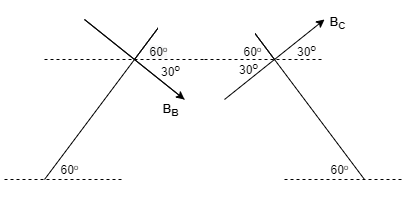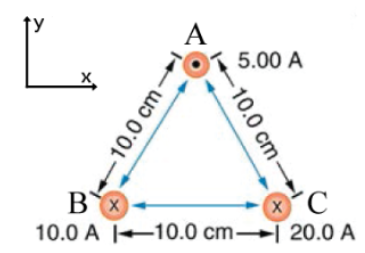# Problem: The figure shows a cross-section of three long straight current-carrying wires. Notice we are using the common convention that crosses denote vectors going into the page and dots denote vectors coming out of the page (a) Find the magnitude of the force per units length, in newtons per meter, on wire A.(b) Find the direction of the force for wire A in degrees. Measure this angle counterclockwise, in degrees, from the positive x-axis.

###### FREE Expert Solution

Magnetic field due to straight currents:

$\overline{){\mathbf{B}}{\mathbf{=}}\frac{{\mathbf{\mu }}_{\mathbf{0}}\mathbf{i}}{\mathbf{2}\mathbf{\pi }\mathbf{r}}}$

Magnetic force on a current-carrying wire:

$\overline{){\mathbf{F}}{\mathbf{=}}{\mathbf{B}}{\mathbf{i}}{\mathbf{L}}{\mathbf{s}}{\mathbf{i}}{\mathbf{n}}{\mathbf{\theta }}}$

(a)

We'll determine the magnetic force at A due to wires B and C. We'll then use the resultant magnetic field to determine the force on A.

The magnetic field around conductors is represented by concentric circles.

The direction at a point is given by the tangent to the circle at that point.

Right-hand rule: Thumb - current. Fingers curl in direction of the magnetic field.Using Right-hand rule, the magnetic field due to conductors B and C is directed in a clockwise direction.

94% (100 ratings)###### Problem Details

The figure shows a cross-section of three long straight current-carrying wires. Notice we are using the common convention that crosses denote vectors going into the page and dots denote vectors coming out of the page(a) Find the magnitude of the force per units length, in newtons per meter, on wire A.
(b) Find the direction of the force for wire A in degrees. Measure this angle counterclockwise, in degrees, from the positive x-axis.